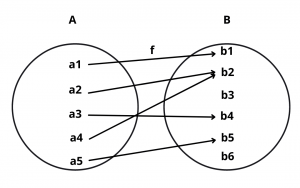# Many One Function – Definition and Examples

Here you will learn what is many one function with definition and examples.

Let’s begin –

## Many One Function

Definition : A function f : A $$\rightarrow$$ B is said to be a many-one function if two or more elements of set A have the same image in B.

Thus, f : A $$\rightarrow$$ B is a many-one if there exist x, y $$\in$$ A such that x $$\ne$$ y but f(x) = f(y).

In other words, f : A $$\rightarrow$$ B is a many-one if it is not a one-one function.

Example : Let f : A $$\rightarrow$$ B and g : X $$\rightarrow$$ Y be two functions represented by the following diagrams :Solution : Clearly, a2 $$\ne$$ a4 but f(a2) = f(a4) and x1 $$\ne$$ x2 but g(x1) = g(x2). So, f and g are many-one.

Example : Let A = {-1, 1, -2, 2} and B = {1, 4, 9, 16}. Consider f : A $$\rightarrow$$ B given by f(x) = $$x^2$$.

Solution : Then, f(-1) = 1, f(1) = 1, f(-2) = 4 and f(2) = 4. Thus, 1 and -1 have the same image. Similarly, 2 and -2 also have the same image. So, f is many-one.